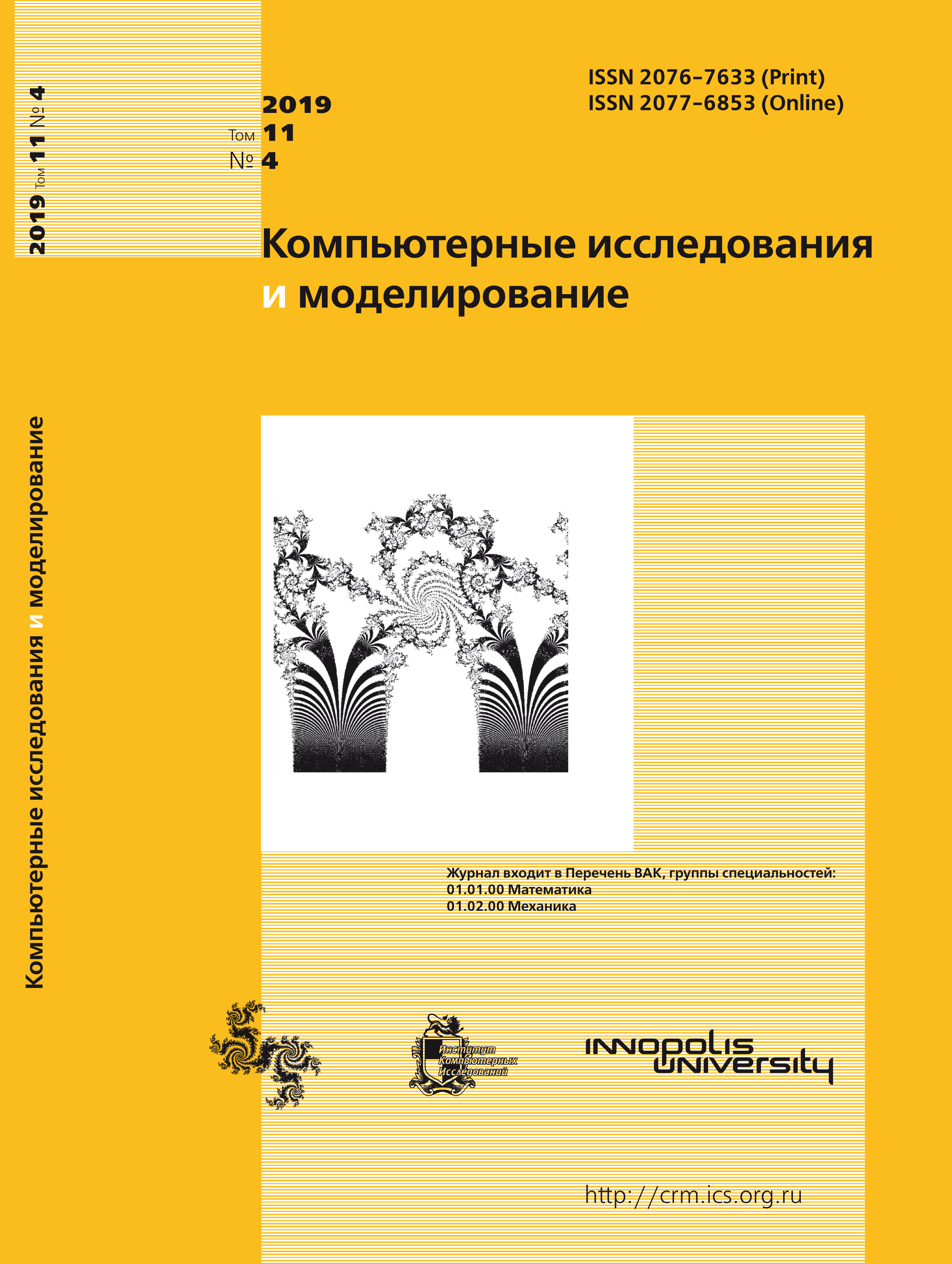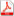Issue 4, 2019 Vol. 11

# All issues

The modeling of nonlinear pulse waves in elastic vessels using the Lattice Boltzmann methodpdf (633K)  / List of references

In the present paper the application of the kinetic methods to the blood flow problems in elastic vessels is studied. The Lattice Boltzmann (LB) kinetic equation is applied. This model describes the discretized in space and time dynamics of particles traveling in a one-dimensional Cartesian lattice. At the limit of the small times between collisions LB models describe hydrodynamic equations which are equivalent to the Navier – Stokes for compressible if the considered flow is slow (small Mach number). If one formally changes in the resulting hydrodynamic equations the variables corresponding to density and sound wave velocity by luminal area and pulse wave velocity then a well-known 1D equations for the blood flow motion in elastic vessels are obtained for a particular case of constant pulse wave speed.

In reality the pulse wave velocity is a function of luminal area. Here an interesting analogy is observed: the equation of state (which defines sound wave velocity) becomes pressure-area relation. Thus, a generalization of the equation of state is needed. This procedure popular in the modeling of non-ideal gas and is performed using an introduction of a virtual force. This allows to model arbitrary pressure-area dependence in the resulting hemodynamic equations.

Two test case problems are considered. In the first problem a propagation of a sole nonlinear pulse wave is studied in the case of the Laplace pressure-area response. In the second problem the pulse wave dynamics is considered for a vessel bifurcation. The results show good precision in comparison with the data from literature.

Keywords: hemodynamics, lattice Boltzmann method, elastic vessels
Citation in English: Ilyin O.V. The modeling of nonlinear pulse waves in elastic vessels using the Lattice Boltzmann method // Computer Research and Modeling, 2019, vol. 11, no. 4, pp. 707-722
Citation in English: Ilyin O.V. The modeling of nonlinear pulse waves in elastic vessels using the Lattice Boltzmann method // Computer Research and Modeling, 2019, vol. 11, no. 4, pp. 707-722
DOI: 10.20537/2076-7633-2019-11-4-707-722
Views (last year): 2.

Full-text version of the journal is also available on the web site of the scientific electronic library eLIBRARY.RU

The journal is included in the Russian Science Citation Index

The journal is included in the List of Russian peer-reviewed journals publishing the main research results of PhD and doctoral dissertations.

International Interdisciplinary Conference "Mathematics. Computing. Education"

The journal is included in the RSCI

Indexed in Scopus